# Maxwell’s inductance bridge

The four arms of Maxwell’s inductance bridge are; Arm AB contains an Inductive coil of inductance L1 having resistance R1. Arms BC and CD contain non-inductive resistances of 200Ω and 100Ω respectively. Arm AD contains standard inductor of inductance L2 and resistance R2. Balance is obtained when L2=50mH and R2=2Ω. Find L1, R1 and Q factor for f= 50 Hz.

L1 =  0.1 H
R1 =  4
Q =  7.854

### Step-by-step explanation: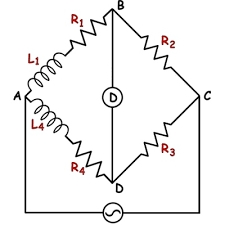Did you find an error or inaccuracy? Feel free to write us. Thank you!Tips to related online calculators
Do you want to convert time units like minutes to seconds?

## Related math problems and questions:

• The coil 2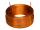The coil of a moving coil voltmeter is 40 mm long and 30 mm wide and has 100 turns on it. The control spring exerts a torque of 240 x 10-6 N-m when the deflection is 100 divisions on full scale. If the flux density of the magnetic field in the air gap is
• Four-digit number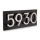For a four-digit number abcd, ab: bc = 1: 3 and bc: cd = 2: 1 (ab, bc and cd are two-digit numbers from digits a, b, c, d). Find this number(s).
• Resistor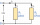Resistor 1 with a resistance of 100 Ohms and resistor 2 with a resistance of 400 Ohms are connected side by side in the circuit. There is a voltage of 80V between the resistor terminals. a) Draw a circuit diagram and write the entered quantities in it b)
• Electronics: Resistors in parallel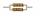From relation for calculating the resistance of parallel combination of resistors: ? Calculate the R, if R1 = 2Ω a R2 = 15Ω
• Coils of transformerThe primary coil of the transformer has 400 turns, a current of 1.5 A passes through it and is connected to a voltage of 220 V. For the secondary coil, find the voltage, current, and a number of turns if the transformation ratio k = 0.1.
• ResistanceDetermine the resistance of the bulb with the current 200 mA and is in a regular lamp (230V).
• Voltmeter range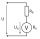We have a voltmeter which in the original set measures voltage to 10V. Calculate the size of the ballast resistor for this voltmeter, if we want to measure the voltage up to 50V. Voltmeter's internal resistance is 2 kiloohm/Volt.
• Coil as a girl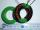The electrical resistance of the copper wire coil is 2.0 ohms. What current runs through the coil when the voltage between the terminals is 3.0 V?
• Reminder and quotientThere are given numbers A = 135, B = 315. Find the smallest natural number R greater than 1 so that the proportions R:A, R:B are with the remainder 1.
• A bottle 2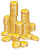A bottle contain 255 coins 1/3 of the coins are £1 cons 110 of the coins are 50p coins the rest of the coins are 20p coins. What is the total value of the coins contained in the bottle.
• Coils of transformerThe primary coil of the transformer has 1100 turns and is connected to a voltage of 220V. How many turns does the secondary coil have when the voltage on it is 55 V? Find the transformation ratio and decide what kind of transformation.
• ResistanceA resistor having an electrical resistance of 1.5 k ohms passes an electrical current of 0.1 A. Calculate what voltage is between the terminals of the resistor.
• Internal anglesThe ABCD is an isosceles trapezoid, which holds: |AB| = 2 |BC| = 2 |CD| = 2 |DA|: On its side BC is a K point such that |BK| = 2 |KC|, on its side CD is the point L such that |CL| = 2 |LD|, and on its side DA the point M is such that | DM | = 2 |MA|. Dete
• RatiosReduce the numbers: 50 in a 1:2 ratio 111 at a ratio of 2:3 70 at 10:50 560 at a ratio of 3:8
• Six paintersSix painters paint an area of 120 m2 in 4 hours. How long does it take for 12 painters to paint 480 m2 with the same performance?
• The tractorThe tractor sows an average of 1.5 ha per hour. In how many hours does it sows a rectangular trapezoid field with the bases of 635m and 554m and a longer arm 207m?
• Marcos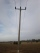Marcos wants to buy grass seed to cover his whole lawn, except for the pool. The pool is 7 1/2 m by 3 1/4 m. Find the area the grass seed needs to cover. Dimensions of the lawn are 50 m x 150 m.